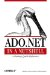# A.1 The SQL Server Provider

The SQL Server provider provides access to a SQL Server database (Version 7.0 or later) through the optimized Tabular Data Stream (TDS) interface (see Table A-1). To connect to the pre-7.0 version of SQL Server, use the OLE DB .NET data provider with the SQL Server OLE DB provider (SQLOLEDB). The connection-specific types are found in the System.Data.SqlClient namespace.

##### Table A-1. SQL Server provider classes

Interface

Implementing class

` IDbConnection `
` SqlConnection `
` IDbCommand `
` SqlCommand `

IDataParameter , IDbDataParameter

` SqlParameter `

` SqlDataReader `
` IDataAdapter, IDbDataAdapter `
` SqlDataAdapter `
` IDbTransaction `
` SqlTransaction `

The SQL Server provider also provides .NET structures that map exactly to SQL Server types in the System.Data.SqlTypes namespace (see Table A-2). You can use SqlDataReader methods such as GetSqlMoney( ) and GetSqlDataTime( ) to retrieve values using these types (as described in Chapter 2).

##### Table A-2. Native SQL Server data types

SQL Server data type

Mapped .NET Type(System namespace)

Underlying .NET type(SqlTypes namespace)

` Binary `
` Byte[] `
` SqlBinary `
` Bigint `
` Int64 `
` SqlInt64 `
` Char `
` String `
` SqlString `
` Datetime `
` Datetime `
` SqlDateTime `
` Decimal `
` Decimal `
` SqlDecimal `
` Float `
` Double `
` SqlDouble `
` Image `
` Byte[] `
` SqlBinary `
` Int `
` Int32 `
` SqlInt32 `
` Money `
` Decimal `
` SqlMoney `
` Nchar `
` String `
` SqlString `
` Ntext `
` String `
` SqlString `
` Nvarchar `
` String `
` SqlString `
` Numeric `
` Decimal `
` SqlDecimal `
` Real `
` Single `
` SqlSingle `
` Smalldatetime `
` Datetime `
` SqlDateTime `
` Smallint `
` Int16 `
` SqlInt16 `
` Smallmoney `
` Decimal `
` SqlMoney `
` sql_variant `
` Object `

System.Object (base class)

` Sysname `
` String `
` SqlString `
` Text `
` String `
` SqlString `
` Timestamp `
` Datetime `
` SqlBinary `
` Tinyint `
` Byte `
` SqlByte `
` Varbinary `
` Byte[] `
` SqlBinary `
` Varchar `
` String `
` SqlString `
` Uniqueidentifier `
` Guid `
` SqlGuid `ADO.NET in a Nutshell
ISBN: 0596003617
EAN: 2147483647
Year: 2005
Pages: 415